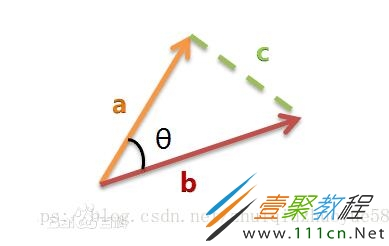• python余弦值反算出角度
2022-02-11 14:44:11

由于工作需要，需要用余弦值（cos）反推出角度是多少。查了半天资料没有解释的很清楚的，索性记录一下，如果能帮助到各位道友，那就更好了！

余弦函数cos 就不用介绍了，说一下反余弦函数（acos）

描述：

acos() 返回x的反余弦弧度值。

用法：

import math

math.acos(x)

注意：acos()是不能直接访问的，需要导入 math 模块，然后通过 math 静态对象调用该方法

返回值：

返回x的反余弦弧度值。

在接着使用math模块中的degrees方法即可得出具体角度值：

import math

print(r)

degree = math.degrees(math.acos(value))
print(degree)开发语言 后端
更多相关内容
• 想了解Python 求向量的余弦值操作的相关内容吗，追求卓越583在本文为您仔细讲解Python向量余弦值的相关知识和一些Code实例，欢迎阅读和指正，我们先划重点：Python,向量,余弦值，下面大家一起来学习吧。1、余弦...

想了解Python 求向量的余弦值操作的相关内容吗，追求卓越583在本文为您仔细讲解Python向量余弦值的相关知识和一些Code实例，欢迎阅读和指正，我们先划重点：Python,向量,余弦值，下面大家一起来学习吧。

1、余弦相似度

余弦相似度衡量的是2个向量间的夹角大小，通过夹角的余弦值表示结果，因此2个向量的余弦相似度为：余弦相似度的取值为[-1,1]，值越大表示越相似。

向量夹角的余弦公式很简单，不在此赘述，直接上代码：

def cosVector(x,y):

if(len(x)!=len(y)):

print('error input,x and y is not in the same space')

return;

result1=0.0;

result2=0.0;

result3=0.0;

for i in range(len(x)):

result1+=x[i]*y[i] #sum(X*Y)

result2+=x[i]**2 #sum(X*X)

result3+=y[i]**2 #sum(Y*Y)

#print(result1)

#print(result2)

#print(result3)

print("result is "+str(result1/((result2*result3)**0.5))) #结果显示

cosVector([2,1],[1,1])

一个计算二维数组余弦值的例子：

#求余弦函数

def cosVector(x,y):

if(len(x)!=len(y)):

print('error input,x and y is not in the same space')

return;

result1=0.0;

result2=0.0;

result3=0.0;

for i in range(len(x)):

result1+=x[i]*y[i] #sum(X*Y)

result2+=x[i]**2 #sum(X*X)

result3+=y[i]**2 #sum(Y*Y)

#print("result is "+str(result1/((result2*result3)**0.5))) #结果显示

return result1/((result2*result3)**0.5)

#print("result is ",cosVector([2,1],[1,1]))

#计算query_output(60,20)和db_output(60,20)的余弦值，用60*1的向量存储

cosResult= [*1 for i in range(60)]

for i in range(60):

cosResult[i]=cosVector(query_output[i], db_output[i])

print(cosResult)

--------------------------------------------------------------------------------------------

#计算query_output和db_output的余弦值，用60*1的向量存储

rows=query_output.shape #行数

cols=query_output.shape #列数

cosResult= [*1 for i in range(rows)]

for i in range(rows):

cosResult[i]=cosVector(query_output[i], db_output[i])

#print(cosResult)

#将结果存入文件中，并且一行一个数字

file=open('cosResult.txt','w')

for i in cosResult:

file.write(str(i).replace('[','').replace(']','')+'\n') #\r\n为换行符

file.close()

补充：python实现余弦近似度

方法一：

def cos(vector1,vector2):

dot_product = 0.0

normA = 0.0

normB = 0.0

for a,b in zip(vector1,vector2):

dot_product += a*b

normA += a**2

normB += b**2

if normA == 0.0 or normB==0.0:

return None

else:

return 0.5 + 0.5 * dot_product / ((normA*normB)**0.5) #归一化 从[-1,1]到[0,1]

方法二：

num = float(A.T * B) #若为行向量则 A * B.T

denom = linalg.norm(A) * linalg.norm(B)

cos = num / denom #余弦值

sim = 0.5 + 0.5 * cos #归一化 从[-1,1]到[0,1]

以上为个人经验，希望能给大家一个参考，也希望大家多多支持。如有错误或未考虑完全的地方，望不吝赐教。

相关文章

展开全文• 本篇文章小编给大家分享一下Python求向量的余弦值操作代码示例，文章代码介绍的很详细，小编觉得挺不错的，现在分享给大家供大家参考，有需要的小伙伴们可以来看看。1、余弦相似度余弦相似度衡量的是2个向量间的夹角...

本篇文章小编给大家分享一下Python求向量的余弦值操作代码示例，文章代码介绍的很详细，小编觉得挺不错的，现在分享给大家供大家参考，有需要的小伙伴们可以来看看。

1、余弦相似度

余弦相似度衡量的是2个向量间的夹角大小，通过夹角的余弦值表示结果，因此2个向量的余弦相似度为：余弦相似度的取值为[-1,1]，值越大表示越相似。

向量夹角的余弦公式很简单，不在此赘述，直接上代码：

def cosVector(x,y):

if(len(x)!=len(y)):

print('error input,x and y is not in the same space')

return;

result1=0.0;

result2=0.0;

result3=0.0;

for i in range(len(x)):

result1+=x[i]*y[i] #sum(X*Y)

result2+=x[i]**2 #sum(X*X)

result3+=y[i]**2 #sum(Y*Y)

#print(result1)

#print(result2)

#print(result3)

print("result is "+str(result1/((result2*result3)**0.5))) #结果显示

cosVector([2,1],[1,1])

一个计算二维数组余弦值的例子：

#求余弦函数

def cosVector(x,y):

if(len(x)!=len(y)):

print('error input,x and y is not in the same space')

return;

result1=0.0;

result2=0.0;

result3=0.0;

for i in range(len(x)):

result1+=x[i]*y[i] #sum(X*Y)

result2+=x[i]**2 #sum(X*X)

result3+=y[i]**2 #sum(Y*Y)

#print("result is "+str(result1/((result2*result3)**0.5))) #结果显示

return result1/((result2*result3)**0.5)

#print("result is ",cosVector([2,1],[1,1]))

#计算query_output(60,20)和db_output(60,20)的余弦值，用60*1的向量存储

cosResult= [*1 for i in range(60)]

for i in range(60):

cosResult[i]=cosVector(query_output[i], db_output[i])

print(cosResult)

--------------------------------------------------------------------------------------------

#计算query_output和db_output的余弦值，用60*1的向量存储

rows=query_output.shape #行数

cols=query_output.shape #列数

cosResult= [*1 for i in range(rows)]

for i in range(rows):

cosResult[i]=cosVector(query_output[i], db_output[i])

#print(cosResult)

#将结果存入文件中，并且一行一个数字

file=open('cosResult.txt','w')

for i in cosResult:

file.write(str(i).replace('[','').replace(']','')+'\n') #\r\n为换行符

file.close()

展开全文• 夹角余弦(Cosine) 也可以叫余弦相似度。 几何中夹角余弦可用来衡量两个向量方向的差异，机器学习中借用这一...求得两个向量的夹角，并得出夹角对应的余弦值，此余弦值就可以用来表征这两个向量的相似性。夹角越小，趋
• I have this array named a of 1242 ... I need to get the cosine value for all the numbers in Python.When I use : cos_ra = math.cos(a) I get an error stating:TypeError: only length-1 arrays can be ...I have this array named a of 1242 numbers. I need to get the cosine value for all the numbers in Python.

When I use : cos_ra = math.cos(a) I get an error stating:

TypeError: only length-1 arrays can be converted to Python scalars

How can I solve this problem??

解决方案

Problem is you're using numpy.math.cos here, which expects you to pass a scalar. Use numpy.cos if you want to apply cos to an iterable.

In : import numpy as np

In : np.cos(np.array([1, 2, 3]))

Out: array([ 0.54030231, -0.41614684, -0.9899925 ])

Error:

In : np.math.cos(np.array([1, 2, 3]))

---------------------------------------------------------------------------

TypeError Traceback (most recent call last)

in ()

----> 1 np.math.cos(np.array([1, 2, 3]))

TypeError: only length-1 arrays can be converted to Python scalars

展开全文• 又名余弦相似度，代码如下：import mathdef cos_dist(a, b):if len(a) != len(b):return Nonepart_up = 0.0a_sq = 0.0b_sq = 0.0for a1, b1 in zip(a,b):part_up += a1*b1a_sq += a1**2b_sq += b1**2part_down = ...
• resemble():'''计算两个句子的相似度：1，将输入的两个句子分词2，求分词后两句子的并集(去重)3，计算两句子各自词频4，求词频向量5，套用余弦定理公式求出相似度余弦值越接近1，就表明夹角越接近0度，也就是两个...
• Pythonic的Python向量夹角余弦值计算 通过公式我们知道，cos⁡(x⃗,y⃗)=x⃗⋅y⃗∣x⃗∣×∣y⃗∣\cos{(\vec{x}, \vec{y})} = \frac{\vec{x}\cdot\vec{y}}{|\vec{x}|\times|\vec{y}|}cos(x,y​)=∣x∣×∣y​∣x⋅y...
• 向量空间夹角余弦值数学公式 欧氏空间中定义了标准内积,就是对应分量相乘之和.这一点也和2,3维空间中内积定义的一样. 那么向量a,b夹角的余弦为： cos=(ab的内积)/(|a||b|) 即：a,b的内积除以它们的模的乘积等于二者...
• 主要介绍了python代码如何实现余弦相似性计算,文中通过示例代码介绍的非常详细，对大家的学习或者工作具有一定的参考学习价值,需要的朋友可以参考下
• 1、余弦相似度余弦相似度衡量的是2个向量间的夹角大小，通过夹角的余弦值表示结果，因此2个向量的余弦相似度为： cosθ=A⋅B||A||∗||B||(1) 余弦相似度的取值为[-1,1]，值越大表示越相似。向量夹角的余弦公式很...
• CosineSimilarity：进行余弦相似度算法比对出来的结果，范围是[-1,1]，比对的结果如果越接近1，表示两者的越相近，越接近-1意味着两者的越相反。 MaxAbsoluteError：进行最大绝对误差算法比对出来的结果，越...
• 背景在计算相似度时，常常用到余弦夹角来判断相似度，Cosine（余弦相似度）取值范围[-1,1]，当两个向量的方向重合时夹角余弦取最大1，当两个向量的方向完全相反夹角余弦取最小值-1，两个方向正交时夹角余弦取值为0...
• ## python余弦相似度

千次阅读 2019-03-26 14:40:04
余弦相似度用向量空间中两个向量夹角的余弦值作为衡量两个个体间差异的大小。余弦值越接近1，就表明夹角越接近0度，也就是两个向量越相似，这就叫"余弦相似性"。 我们知道，对于两个向量，如果他们之间的夹角越小，...
• 今天打算通过绘制正弦和余弦函数，从默认的设置开始，一步一步地调整改进，让它变得好看，变成我们初高中学习过的图象那样。通过这个过程来学习如何进行对图表的一些元素的进行调整。 01. 简单绘图 matplotlib有一套...
• 分词A：西米／喜欢／健身B：超超／不／喜欢／健身，喜欢／打／游戏step2:列出两个句子的并集西米／喜欢／健身／超超／不／打／游戏step3:计算词频向量A：[1,1,1,0,0,0,0]B：[0,1,1,1,1,1,1]step4:计算余弦值余弦值越...
• 向量余弦值pythonPrerequisite: 先决条件： Defining a Vector定义向量 Defining a Matrix定义矩阵 Numpy is the library of function that helps to construct or manipulate matrices and vectors. The function...
• ## Python计算余弦相似度

万次阅读 多人点赞 2020-08-09 10:51:39
余弦相似度常用在文本分类、图片分类等应用中，来计算两个文本或两个图像之间的相似度。 本文主要介绍通过Python计算两个向量的余弦相似度
• 余弦相似度，又称为余弦相似性，是通过计算两个向量的夹角余弦值来评估他们的相似度。对于两个向量，可以想象成空间中的两条线段，都是从原点（[0, 0, ...]）出发，指向不同的方向。两条线段之间形成一个夹角：如果...
• 描述cos() 返回x的弧度的余弦值。语法以下是 cos() 方法的语法:import mathmath.cos(x)注意：cos()是不能直接访问的，需要导入 math 模块，然后通过 math 静态对象调用该方法。参数x -- 一个数值。返回值返回x的弧度...
• 本文旨在对两个未知类型的文本进行余弦相似度分析，判断哪个文本属于军事类理论知识 对于二维空间，根据空间向量点积公式：假设...余弦值的范围在[-1,1]之间，值越趋近于1，代表两个向量的方向越接近（文档越相似）；接
• 主要介绍了Python使用matplotlib绘制正弦和余弦曲线的方法,结合实例形式分析了Python调用matplotlib库进行图形绘制想具体操作技巧,需要的朋友可以参考下
• 在我们计算相似度时，常常用到余弦夹角来判断两个向量或者矩阵之间的相似度，Cosine（余弦相似度）取值范围[-1,1]，当两个向量的方向重合时夹角余弦取最大1，当两个向量的方向完全相反夹角余弦取最小值-1，两个...
• 当前实现了十二种算法（包括Levenshtein编辑距离和同级，Jaro-Winkler，最长公共子序列，余弦相似性等）。 查看下面的摘要表以获取完整列表... 下载 从pypi： # pip install strsim # deprecated, do not use this...
• cos()方法返回x弧度的余弦值。 语法 以下是cos()方法的语法： cos(x) 注意：此函数是无法直接访问的，所以我们需要导入math模块，然后需要用math的静态对象来调用这个函数。 参数 x — 这必须是一个数值 返回值 此...
• 如果可以使用SciPy,可以使用spatial.distance中的余弦值：如果您不能使用SciPy,您可以尝试通过重写Python来获得一个小的加速(编辑：但是没有像我以前想像的那样工作,见下文).from itertools import izipfrom math ......